Points collinear

Show that the point A(-1,3), B(3,2), C(11,0) are col-linear.

Result

x =  1

Solution:Leave us a comment of example and its solution (i.e. if it is still somewhat unclear...):Be the first to comment!To solve this verbal math problem are needed these knowledge from mathematics:

For Basic calculations in analytic geometry is helpful line slope calculator. From coordinates of two points in the plane it calculate slope, normal and parametric line equation(s), slope, directional angle, direction vector, the length of segment, intersections the coordinate axes etc. Two vectors given by its magnitudes and by included angle can be added by our vector sum calculator.

Next similar examples:

1. Vector - basic operationsThere are given points A [-9; -2] B [2; 16] C [16; -2] and D [12; 18] a. Determine the coordinates of the vectors u=AB v=CD s=DB b. Calculate the sum of the vectors u + v c. Calculate difference of vectors u-v d. Determine the coordinates of the vector w.
2. Symmetry by plane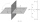Determine the coordinates of a image of point A (3, -4, -6) at a symmetry that is determined by the plane x-y-4z-13 = 0
3. LineLine p passing through A[-10, 6] and has direction vector v=(3, 2). Is point B[7, 30] on the line p?
4. Virus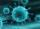We have a virus that lives one hour. Every half hour produce two child viruses. What will be the living population of the virus after 3.5 hours?
5. SequenceBetween numbers 1 and 53 insert n members of the arithmetic sequence that its sum is 702.
6. LineIt is true that the lines that do not intersect are parallel?Given that P = (5, 8) and Q = (6, 9), find the component form and magnitude of vector PQ.
8. Scalar product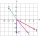Calculate the scalar product of two vectors: (2.5) (-1, -4)
9. Scalar dot productCalculate u.v if |u| = 5, |v| = 2 and when angle between the vectors u, v is: a) 60° b) 45° c) 120°
10. Committees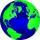How many different committees of 6 people can be formed from a class of 30 students?
11. VectorDetermine coordinates of the vector u=CD if C[19;-7], D[-16,-5].
12. Parametric equationFind the parametric equation of a line with y-intercept (0,-4) and a slope of -2.
13. Theorem proveWe want to prove the sentence: If the natural number n is divisible by six, then n is divisible by three. From what assumption we started?
14. Coordinates of vector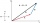Determine the coordinate of a vector u=CD if C(19;-7) and D(-16;-5)
15. Fish tankA fish tank at a pet store has 8 zebra fish. In how many different ways can George choose 2 zebra fish to buy?
16. Six termsFind the first six terms of the sequence a1 = -3, an = 2 * an-1
17. DiscriminantDetermine the discriminant of the equation: ?